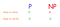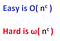Easily Understand NP vs P Problem

# P = NP ?

This is one of the most famous problems in computer science. A correct solution to the problem does P=NP, will get you \$1 million USD. This problem asks whether or not for all problems that can be verified in polynomial time, can they also be solved in polynomial time ? That is to say can every solved problem whose answer can be checked quickly by a computer also be quickly solved by a computer? -(Wikipedia) This problem is apart of the Millennium prize problems, and was conceived in 1971.Fig 1: A picture showing ‘P-problems’ are easily solved and easy to verify, while ‘NP-problems’ are easy to verify but have not been proven to be easily solved.

P problems are fast for computers to solve, and so are considered “easy” or problems that can be solved in polynomial time. These are problems like multiplication or alphabetizing a list of names. NP problems are fast (and so “easy”) for a computer to check, meaning they can be checked in polynomial time, but are not necessarily easy to solve. These problems include job scheduling, vehicle routing (TSP), circuit design, Sudoku, and database problems (feedback vertex set), and the Hamiltonian Path Problem which basically says “ given N cities to visit, how can a person visit every city without visiting a city twice. Finding prime numbers was once thought to only be in NP class and not P, but was later found to be a P problem as well.Asymptotics showing easy problems belong to Big O of n^c and hard problems belong to little Omega of n^c. Note: ‘c’ is a constant and ’n’ is a variable

If all NP problems are really in P then many important puzzles/problems like curing cancer ( protein folding), economics (efficient markets), and public key encryption (which we use for online banking and credit cards would be easy to crack).

Most computer scientists and mathematicians believe P does not equal NP. So does being able to quickly recognize a correct answer mean there's also a quick way to find them ? I don’t know, but please comment if you have the answer !

Thanks for reading this article I hope its helpful to you all ! Keep up the learning, and if you would like more computer science, programming and algorithm analysis videos please visit and subscribe to my YouTube channels (randerson112358 & compsci112358 )

# Check Out the following for content / videos on Computer Science, Algorithm Analysis, Programming and Logic:

compsci112358:

Video Tutorials on Recurrence Relation:

Video Tutorial on Algorithm Analysis:
https://www.udemy.com/algorithm-analysis/

Written by